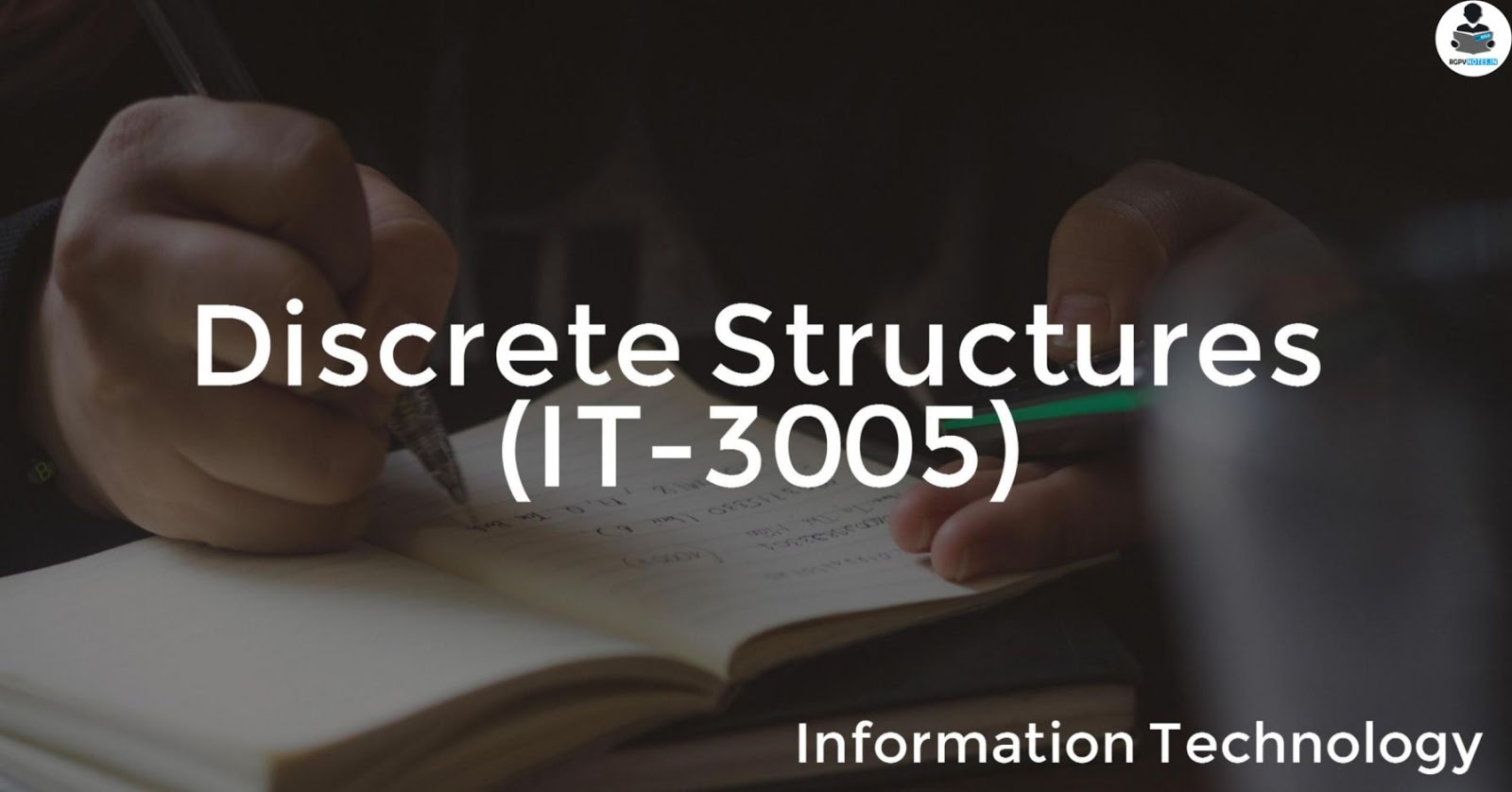### Discrete Structures (IT-3005)## Objectives

The main objectives of this course are:
1. To introduce students with sets, relations, functions, graph, and probability.
2. Students can perform set operation and solve logical reasoning and verify the correctness of logical statement.
3. They can apply the properties of relations and find partially ordered set and lattices.

## Syllabus

UNIT 1:Set Theory, Relation, Function, Theorem Proving Techniques : Set Theory: Definition of sets, countable and uncountable sets, Venn Diagrams, proofs of some general identities on sets Relation: Definition, types of relation, composition of relations, Pictorial representation of relation, Equivalence relation, Partial ordering relation, Job Scheduling problem Function: Definition, type of functions, one to one, into and onto function, inverse function, composition of functions, recursively defined functions, pigeonhole principle. Theorem proving Techniques: Mathematical induction, Proof by contradiction.

UNIT 2: Algebraic Structures: Definition, Properties, types: Semi Groups, Monoid, Groups, Abelian group, properties of groups, Subgroup, cyclic groups, Normal subgroup, Homomorphism and isomorphism of Groups, example and standard results, Rings and Fields: definition and standard results.

UNIT 3: Propositional Logic: Proposition, First order logic, Basic logical operation, truth tables, tautologies, Contradictions, Algebra of Proposition, logical implications, logical equivalence, predicates, Normal Forms, Universal and existential quantifiers. Introduction to finite state machine Finite state machines as models of physical system equivalence machines, Finite state machines as language recognizers

UNIT 4: Graph Theory: Introduction and basic terminology of graphs, Planer graphs, Multigraphs and weighted graphs, Isomorphic graphs, Paths, Cycles and connectivity, Shortest path in weighted graph, Introduction to Eulerian paths and circuits, Hamiltonian paths and circuits, Graph coloring, chromatic number, Isomorphism and Homomorphism of graphs.

UNIT 5: Posets, Hasse Diagram and Lattices: Introduction, ordered set, Hasse diagram of partially, ordered set, isomorphic ordered set, well ordered set, properties of Lattices, bounded and complemented lattices. Combinatorics: Introduction, Permutation and combination, Binomial Theorem, Recurrence Relation and Generating Function: Introduction to Recurrence Relation and Recursive algorithms , Linear recurrence relations with constant coefficients, Homogeneous solutions, Particular solutions, Total solutions , Generating functions , Solution by method of generating functions.

• Unit 1
• Unit 2
• Unit 3
• Unit 4
• Unit 5

## Outcomes

On completion of the course:
1. Students will be able to understand the notion of mathematical thinking, and algorithmic thinking and be able to apply them in problem solving such as formal specification, verification, and basic concepts of set theory.
2. Students understand the basic principle of Boolean algebra, logic and set theory.
3. Be able to construct simple mathematical proof and possess the ability to verify them.

## References

1. C.L.Liu” Elements of Discrere Mathematics” TMH.
2. Lipschutz, “Discrete mathematics (Schaum)”,TMH.
3. U.S Gupta “ Discrete Mathematical Structures” Pearson.
4. S. Santha,” Discrete Mathematics with Combinatorics and graph theory”, Cengage Learning.
5. Dr.Sukhendu. Dey “ Graph Theory With Applications” Shroff Publishers